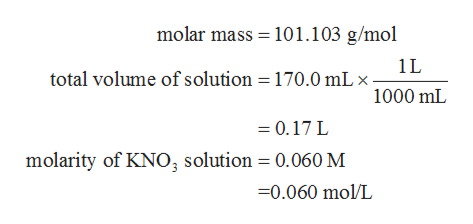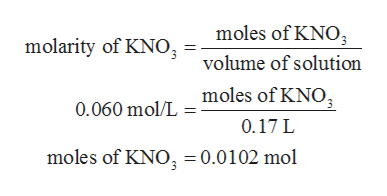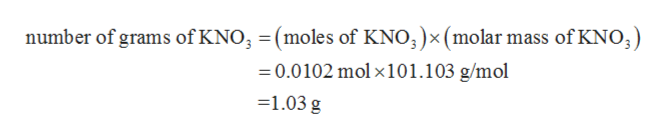# How many grams of KNO3 (molar mass = 101.103 g/mol) should be used to make 170.0 mL of 0.060 M solution?

Question
32 views

How many grams of KNO3 (molar mass = 101.103 g/mol) should be used to make 170.0 mL of 0.060 M solution?

check_circle

Step 1

The values are given as follows:help_outlineImage Transcriptionclosemolar mass 101.103 g/mol 1L total volume of solution 170.0 mL 1000 mL = 0.17 L molarity of KNO, solution 0.060M -0.060 mol/L fullscreen
Step 2

The number of moles of KNO3 can be calculated as follows:help_outlineImage Transcriptionclosemoles of KNO molarity of KNO volume of solution moles of KNO, 0.060 mol/L 0.17 L moles of KNO = 0.0102 mol fullscreen
Step 3

The required number of grams of KNO3 can be determined...help_outlineImage Transcriptionclosenumber of grams of KNO (moles of KNO;)x (molar mass of KNO; 0.0102 mol x101.103 g/mol =1.03 g fullscreen

### Want to see the full answer?

See Solution

#### Want to see this answer and more?

Solutions are written by subject experts who are available 24/7. Questions are typically answered within 1 hour.*

See Solution
*Response times may vary by subject and question.
Tagged in

### Chemistry